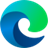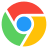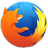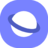# rotate3d() CSS Function

### Description

The CSS `rotate3d()` function is used to rotate an element around a fixed axis in 3D space. It takes four parameters:

• `x`: The x-coordinate of the axis of rotation.
• `y`: The y-coordinate of the axis of rotation.
• `z`: The z-coordinate of the axis of rotation.
• `angle`: The angle of rotation in degrees.

A positive angle results in a clockwise rotation, while a negative angle results in a counter-clockwise rotation.

The `rotate3d()` function can be used to create a variety of 3D effects, such as rotating a cube, spinning a sphere, or flipping a card over. It is often used in conjunction with other CSS transform functions, such as `translate3d()` and `scale3d()`, to create complex and dynamic 3D animations.

Here is an example of how to use the `rotate3d()` function to rotate a cube:

``````.cube {
transform: rotate3d(1, 1, 0, 45deg);
}
``````

This will rotate the cube 45 degrees around an axis that passes through the center of the cube and is aligned with the x- and y-axes.

### Syntax

`transform: rotate3d( <numberX> , <numberY> , <numberZ> , <angle> );`

### Values

• lt;numberX>Is a lt;number> describing the x-coordinate of the vector denoting the axis of rotation which could between 0 and 1.
• lt;numberY>Is a lt;number> describing the y-coordinate of the vector denoting the axis of rotation which could between 0 and 1.
• lt;numberZ>Is a lt;number> describing the z-coordinate of the vector denoting the axis of rotation which could between 0 and 1.
• lt;angle>Is an lt;angle> representing the angle of the rotation. A positive angle denotes a clockwise rotation, a negative angle a counter-clockwise one.

### Example

``````<div class="normal rotated">Rotated</div>
<div class="normal">Not rotated</div>``````
``````.normal {
margin: 20px;
width: 120px;
background: #546D62;
color: white;
font-family: sans-serif;
}
.rotated {
transform: rotate3d(1, 3, 1, 60deg);
}``````

### Browser Support

The following table will show you the current browser support for the CSS `rotate3d()` function.

 Desktop12 12 10 15 4
 Tablets / Mobile18 10 14 3.2 1 3

Last updated by CSSPortal on: 7th October 2023